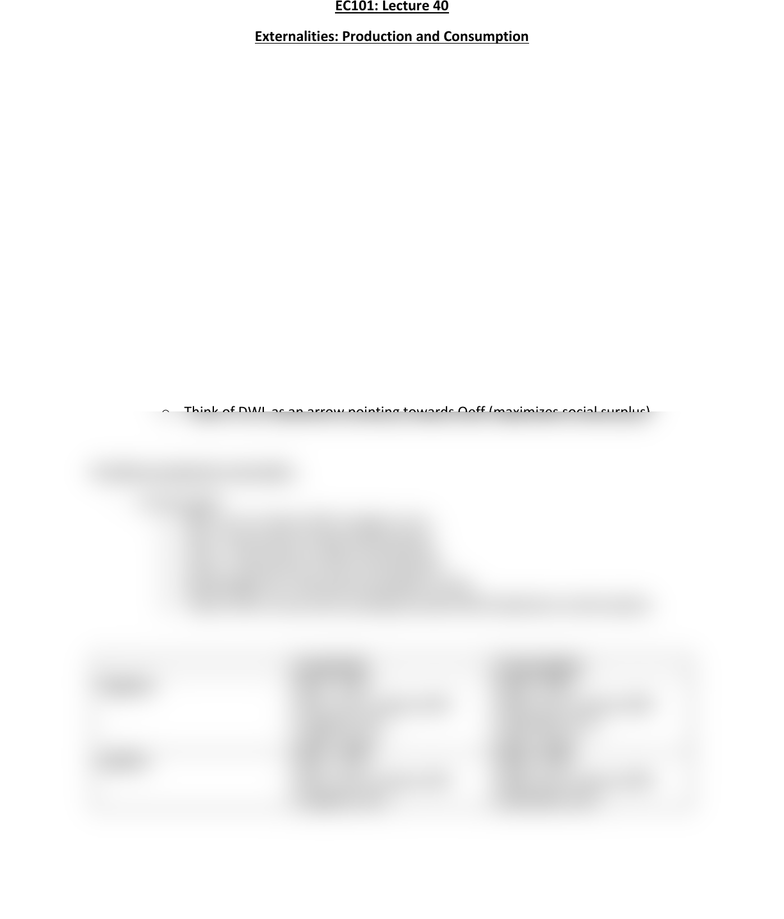Get 2 days of unlimited access
Class Notes (1,000,000)
US (420,000)
BU (8,000)
CAS EC (600)
Lecture 40

# CAS EC 101 Lecture Notes - Lecture 40: Deadweight Loss, Demand Curve, ExternalityPremium

Department
Economics
Course Code
CAS EC 101
Professor
Bruce Watson
Lecture
40

This preview shows half of the first page. to view the full 2 pages of the document.EC101: Lecture 40
Externalities: Production and Consumption
Definitions
- MSC = margina societal cost
- MPC = marginal private cost
- MSB = marginal societal benefit
- MPB = marginal private benefit
- DWL = deadweight loss
- Qeff = most efficient quantity
- Qmkt = quantity that is actually sold
Negative Production externality
- On the graph:
o MSC curve is above MPC (Supply) curve
o Qeff = intersection of MSC and Demand
o Qmkt = intersection of MPC and Demand
o Deadweight loss: they get the quantity wrong
o Think of DWL as an arrow pointing towards Qeff (maximizes social surplus)
Prositive production externality
- On the graph
o MSC curve is below MPC (supply) curve
o Qeff = intersection of MSC and Demand
o Qmkt = intersection of MPC and Demand
o Deadweight loss: they get the quantity wrong
o Think of DWL as an arrow pointing towards Qeff (maximizes social surplus)
production
Consumption
Negative
MSC > MPC
MSC curve is above MPC
(supply) curve
Qeff < Qmkt
MSB < MPB
MSB curve is below MPB
(demand) curve
Qeff < Qmkt
positive
MSC < MPC
MSC curve is below MPC
(supply) curve
MSB > MPB
MSB curve is above MPB
(demand) curve
###### You're Reading a Preview

Unlock to view full version

Subscribers Only